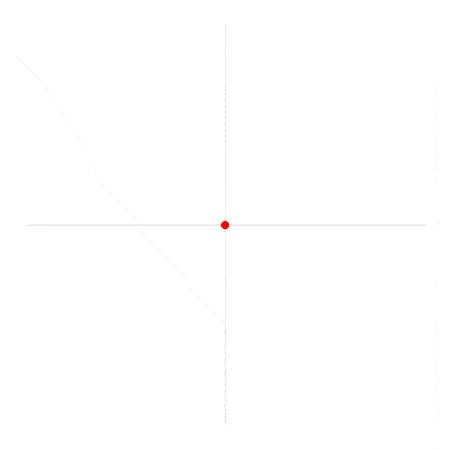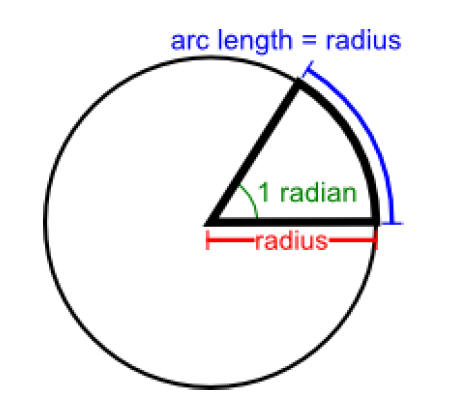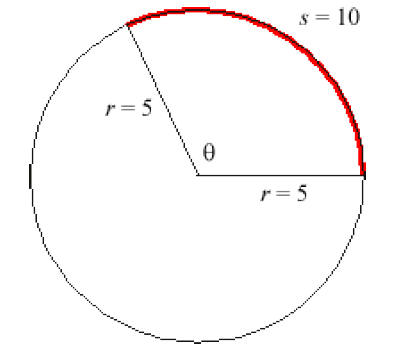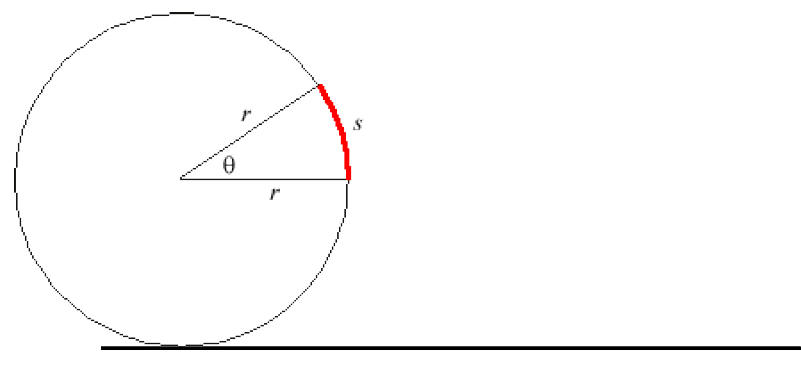Rotational Kinematics

A rolling object can have 2 motions:

[ Larger ]

b) Translational  Motion - (m/s)

Movement of object's center of mass from one (x,y) position to another (x,y) postionIn order to understand the physics of a rolling object, it's helpful to measure it rotational kinematics in terms of radians.a) When a round object rotates 1 radian, it has travelled one radii

b) One full rotation of a sphere = 360 degrees or .......

[ Larger ]

57 degrees

Equation:

 q = d/r

And d =

 d = qrEx) How many radians does the arc s represent?2

Ex 1) A wheel with a radius of 2.0 m rolls 5.0 m along the floor.

Find the number of radians rotated through.

q = d/rEx 2) How many radians are subtended by a 0.10 m arc of a circle of radius of 0.40 m?

 d = qr

Ex 3) How many degrees are subtended by a 0.10 m arc of a circle of radius of 0.40 m?

14 degrees

II. Angular Velocity:

a) defined - How fast something spins

c) Linear velocity (v) and Angular velocity w

Formula:

 v = wr

v/r = w

Ex 4) A ball is rolling along the ground at 5.0 m/s.

If the ball's radius is .50 m, what is its angular velocity?v = wr

5 m/s = w(.50m)

d) Rotating object's period - time
for one complete ........  rotation

 T = 2p/w

 T = 1/f

 f = w/2p

Ex 5) Find the period for a rolling object with a rotational velocity of 10. rad/sec.

Find period.T = 2p/w

T = .63 sec

f = 1.6 Hz

III. Angular Acceleration

Review: Relating Linear motion to Rotational motion

d = rq

q = distance rotated in radians

v = rw

w - angular velocity

So … a = ra

Since a = v2/r

then ..

a = r2w2/r

a = rw2

Ex 6) A pool ball with a radius of 4.0 cm accelerates from rest to 5.0 m/s in .1 seconds.

Find its angular acceleration.

Since all the info is linear, find a then convert

a = Dw/Dt

a = 50. m/s

a = r
a

a = 1.3 x 103 rad/s2

Match the rotational variable with its linear kinematic variablea =   w = q =

d = ____ v = _____ a = _____

 Linear Kinematics / Rotational Kinematics Conversion Linear Kinematics Rotational Kinematics a = DV/Dt Vf = Vi + t V = Dd/Dt Vf2 = Vf2 + 2aDd Dd = Vit + ½ at2

Ex 7) A ball with a radius of .10 m starts from rest and accelerates down a 10. m incline and attains a velocity of 8.0 m/s.

Find it’s ANGULAR acceleration.

Find a then a

a = 3.2 m/s2

a = raProblems